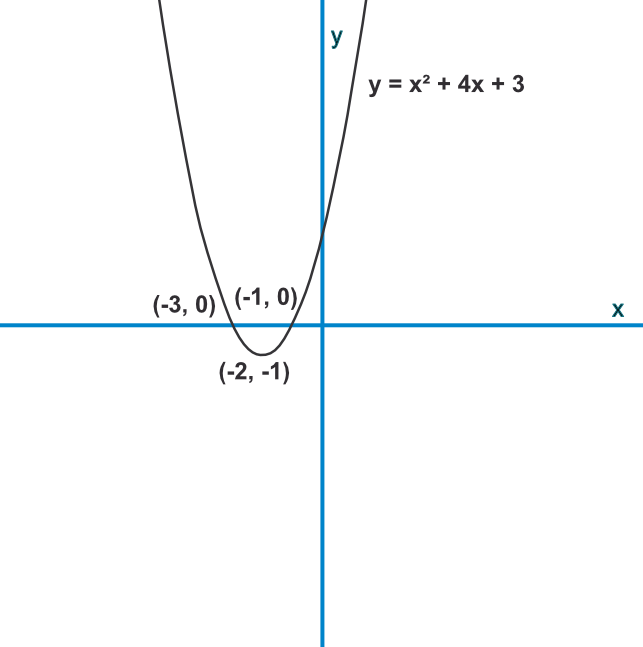Working with Roots in Algebra

## Working with Roots in Algebra

There may be 0, 1 or 2 roots for a quadratic. A root is found for x when f(x)=0. This can be seen graphically when the curve crosses y=0. If the curve does not cross y=0 then there are no real roots for the function.

The roots can be obtained by:

• rearranging as factors; (Factorising)

• using the quadratic formula x = frac(-b +- √(b^2 - 4ac))(2a); (Quadratic Formula)

• completing the square; (Completing the Square)

• deducing iteratively (for a positive square root)

• and for an approximate solution: drawing a graph of the function.

## Example 1

Sketch the graph of x^2 +4x + 3 showing the coordinates of the roots and the turning point.

Factorise the equation to (x+3) and (x+1). This gives the roots as x=-3 and x=-1

The turning point is given using -frac(b)(2a) = frac(-4)(2(1)) = -2 This is the x value, substitute into the original equation for (-2)^2 +4(-2)+ 3 = 4 - 8 + 3 = -1

Roots are (-3,0) and (-1, 0); turning point is (-2,-1)## Example 2

The roots of a quadratic are -5 and 7. The turning point is (1, -36). The coefficient of the squared term is 1. What is the quadratic?

The roots give the factors of the expression (x + 5)(x - 7)

Multiply that out to give x^2 - 2x - 35

Check with the turning point: -36 = (1)^2 - 2(1) - 35

Answer: x^2 - 2x -35